Next: Worked example 10.5: Rod Up: Statics Previous: Worked example 10.3: Leaning

## Worked example 10.4: Truck crossing a bridge

Question: A truck of mass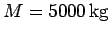is crossing a uniform horizontal bridge of mass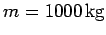and length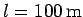. The bridge is supported at its two end-points. What are the reactions at these supports when the truck is one third of the way across the bridge?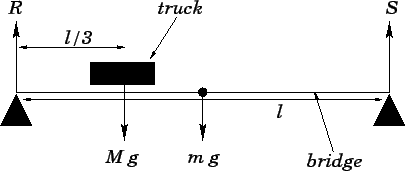Answer: Letandbe the reactions at the bridge supports. Here,is the reaction at the support closest to the truck. Setting the net vertical force acting on the bridge to zero, we obtain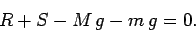Setting the torque acting on the bridge about the left-most support to zero, we get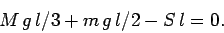Here, we have made use of the fact that centre of mass of the bridge lies at its mid-point. It follows from the above two equations that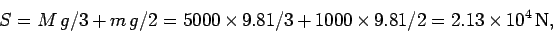and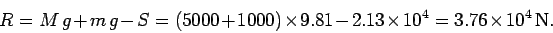Next: Worked example 10.5: Rod Up: Statics Previous: Worked example 10.3: Leaning
Richard Fitzpatrick 2006-02-02